MORE IN Graph Theory and its Applications
VTU Computer Science (Semester 4)
Graph Theory and its Applications
June 2015
Total marks: --
Total time: --
INSTRUCTIONS
(1) Assume appropriate data and state your reasons
(2) Marks are given to the right of every question
(3) Draw neat diagrams wherever necessary

1 (a) For the following graph determine,
i) A walk from b to d that is not trail
ii) A b-d trail that is not path
iii) A path from b to d
iv) A closed walk from b to b that is not a circuit
v) A circuit from b to b that is not a cycle
vi) A cycle from b to b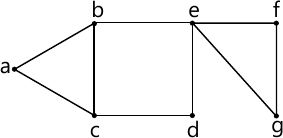6 M
1 (b) Define subgraph, spanning subgraph , induced subgraph and complete graph with example
7 M
1 (c) Prove that the undirected graph G=(V,E) has an Euler circuit if and only if G is connected and every vertex in G has even degree
7 M

2 (a) Define planar graph and prove that the following Petersen graph is nonplanar using Kuratowski's theorem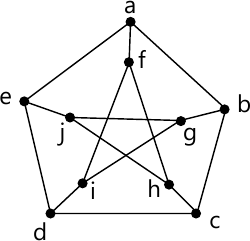6 M
2 (b) Prove that in a complete graph with n-vertices, where n is an add number ≥ 3, there are (n-1)/2 edge-disjoint Hamiltonian cycles
7 M
2 (c) Find the chromatic polynomial for the following graph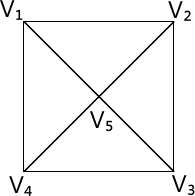7 M

3 (a) Prove that in every tree T=(V,E)$\left | V \right |=\left | E \right |+1$
6 M
3 (b) If T1=(V1,E1) and T2=(V2,E2) be two trees where $\left | E_{1} \right |=17$ and $\left | V_{2} \right |=2\left | V_{1} \right |$, then find $\left | V_{1} \right |,\left [ V_{2} \right ] and \left | E_{1} \right |$
ii) Let F2=(V2,E2) is a forest with $\left | V_{2} \right |=62 and \left | E_{2} \right |=51$ , how many trees determine F2
iii) Let F1=(V1,E) be a forest of 7 trees where $\left | E_{1} \right |=40$ what is $\left | V_{1} \right |?$
7 M
3 (c) Construct an optional prefix code for the symbols a,o,q,u,y,z that occur with frequencies 20,28,4,17,12,7 respectively
7 M

4 (a) Using the Kruskal's algorithm, find a minimal spanning three of the following weighted graphs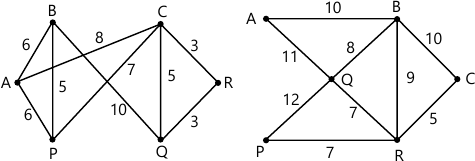8 M
4 (b) Using the Dijkstra's algorithm obtain the shortest path from vertex 1 to each of the other vertices in the following graph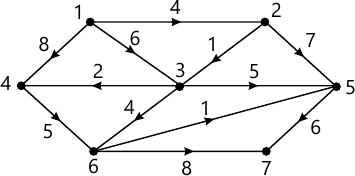7 M
4 (c) Prove that in bipartite graph G(V1,V2,E) if there is positive integer M such that the degree of every vertex in V1≥M≥ the degree of every vertex in V2 then there exists a complete matching from V1 to V 2
7 M

5 (a) i) How many arrangements all there for all letters in the word SOCIOLOGICAL?
ii) In how many of these arrangements, A and G are adjacent?
iii) In how many of these arrangements, all the vowels are adjacent>
6 M
5 (b) Determine to co-efficient of :
i) x9y3 in the expansion of (2x-3y)12
ii) x-y-z2 in the expansion of (2x-y-z)4
iii) x2-y2-z3 in the expansion of (3x-2y-4z)7
7 M
5 (c) Determine the number of integer solutions for :x1+x2+x3+x4+x5<40,
where:
i) xi≥0, 1≤i≤5
ii)xi≥-3,1≤i≤5
7 M

6 (a) Find the number of integers between 1 to 10,000 inclusive, which are divisible by none of 5,6 or 8
6 M
6 (b) Determine in how many ways can the letter in the word ARRANGEMENT be arranged so that there are exactly two pairs of consecutive identical letters
7 M
6 (c) i) Find the rook polynomial for the shaded chessboard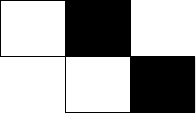ii) Let A={1,2,3,4} and B={u,v,w,x,y,z}. How many one to one functions f:A → B satisfy none of the following conditions:
C1:f(1)=u or v; C2:f(2)=w; C3:f(3)=W or x; C4 : f(4)=x,y or z

7 M

7 (a) Find the coefficient of x15in $\dfrac{(1+x)^{4}}{(1-x)^{4}}$
6 M
7 (b) A ship carries 48 flags, 12 each of the colours red, white, blue and black. Twelve of these flags are placed on an vertical pole in order to communicate a signal to other ships. Determine, how many of these signals have at least three white flags or no white flags at all
7 M
7 (c) Find the formula to express : 02+12+22+--------+n2 as a function of n using summation on operator
7 M

8 (a) Solve the recurrence relation Fn+2=Fn+1+Fn where n ≥ 0 and F0=0 and F1=1
6 M
8 (b) i) A bank pay 6%interest compounded quarterly. If Laura inverts \$ 100 then how many months must she wait for her money to double?
ii) The number of bacteria in culture is 1000 and this number increase 250% every 2 hours. Use a recurrence relation to determine the number to bacteria present after one day.
7 M
8 (c) Solve the recurrence relation :an+2-5a+6an=2, n≥0, a0=3, a1=7 using method of generating functions
7 M

More question papers from Graph Theory and its Applications